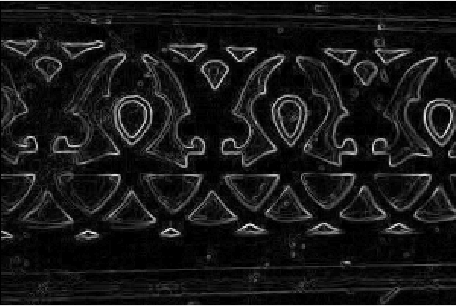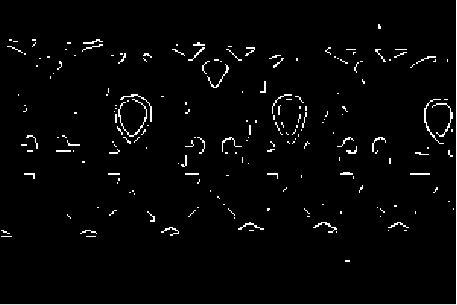# MATLAB – Image Edge Detection using Robert Operator from Scratch

Robert Operator: This gradient-based operator computes the sum of squares of the differences between diagonally adjacent pixels in an image through discrete differentiation. Then the gradient approximation is made. It uses the following 2 x 2 kernels or masks –Approach:

Step 1: Input – Read an image
Step 2: Convert the true-color RGB image to the grayscale image
Step 3: Convert the image to double
Step 4: Pre-allocate the filtered_image matrix with zeros
Step 5: Define Robert Operator Mask
Step 6: Edge Detection Process (Compute Gradient approximation and magnitude of vector)
Step 7: Display the filtered image
Step 8: Thresholding on the filtered image
Step 9: Display the edge-detected image

Implementation in MATLAB:

 % MATLAB Code | Robert Operator from Scratch     % Read Input Image  input_image = imread('[name of input image file].[file format]');     % Displaying Input Image  input_image = uint8(input_image);  figure, imshow(input_image); title('Input Image');     % Convert the truecolor RGB image to the grayscale image  input_image = rgb2gray(input_image);     % Convert the image to double  input_image = double(input_image);     % Pre-allocate the filtered_image matrix with zeros  filtered_image = zeros(size(input_image));     % Robert Operator Mask  Mx = [1 0; 0 -1];  My = [0 1; -1 0];     % Edge Detection Process  % When i = 1 and j = 1, then filtered_image pixel    % position will be filtered_image(1, 1)  % The mask is of 2x2, so we need to traverse   % to filtered_image(size(input_image, 1) - 1  %, size(input_image, 2) - 1)  for i = 1:size(input_image, 1) - 1      for j = 1:size(input_image, 2) - 1             % Gradient approximations          Gx = sum(sum(Mx.*input_image(i:i+1, j:j+1)));          Gy = sum(sum(My.*input_image(i:i+1, j:j+1)));                            % Calculate magnitude of vector          filtered_image(i, j) = sqrt(Gx.^2 + Gy.^2);                end end    % Displaying Filtered Image  filtered_image = uint8(filtered_image);  figure, imshow(filtered_image); title('Filtered Image');     % Define a threshold value  thresholdValue = 100; % varies between [0 255]  output_image = max(filtered_image, thresholdValue);  output_image(output_image == round(thresholdValue)) = 0;     % Displaying Output Image  output_image = im2bw(output_image);  figure, imshow(output_image); title('Edge Detected Image');

Input Image –Filtered Image:Edge Detected Image:1. Detection of edges and orientation are very easy
2. Diagonal direction points are preserved

Limitations:

1. Very sensitive to noise
2. Not very accurate in edge detection

My Personal Notes arrow_drop_upCheck out this Author's contributed articles.

If you like GeeksforGeeks and would like to contribute, you can also write an article using contribute.geeksforgeeks.org or mail your article to contribute@geeksforgeeks.org. See your article appearing on the GeeksforGeeks main page and help other Geeks.

Please Improve this article if you find anything incorrect by clicking on the "Improve Article" button below.

Article Tags :

Be the First to upvote.

Please write to us at contribute@geeksforgeeks.org to report any issue with the above content.